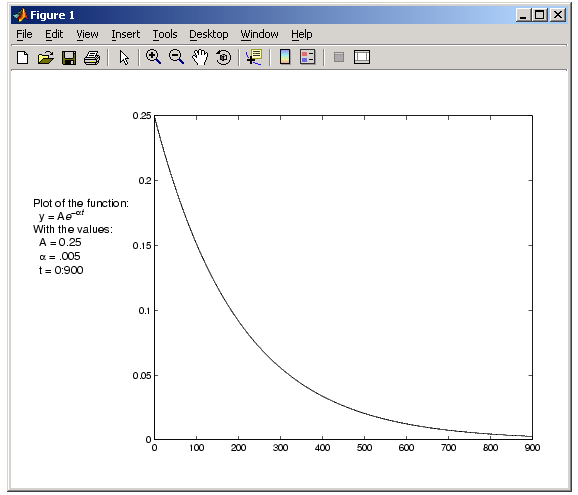GraphicsPlacing Text Outside the Axes

MATLAB always displays text objects within an axes. If you want to create a graph and provide a description of the information alongside the graph, you must create another axes to position the text. If you create an axes that is the same size as the figure and then create a smaller axes to draw the graph, you can then display text anywhere independently of the graph.

For example, define two axes.

• h = axes('Position',[0 0 1 1],'Visible','off');
axes('Position',[.25 .1 .7 .8])


Because the axes units are normalized to the figure, specifying the Position as [0 0 1 1] creates an axes that encompasses the entire window.

Now plot some data in the current axes. The last axes created is the current axes, so MATLAB directs graphics output there.

• t = 0:900;
plot(t,0.25*exp(-0.005*t))


Define the text and display it in the full-window axes.

• str(1) = {'Plot of the function:'};
str(2) = {' y = A{\ite}^{-\alpha{\itt}}'};
str(3) = {'With the values:'};
str(3) = {' A = 0.25'};
str(4) = {' \alpha = .005'};
str(5) = {' t = 0:900'};
set(gcf,'CurrentAxes',h)
text(.025,.6,str,'FontSize',12)Multiple Axes per Figure Multiple Axes for Different Scaling© 1994-2005 The MathWorks, Inc.